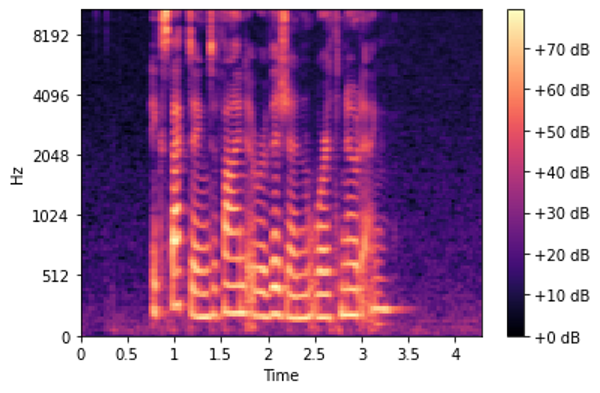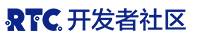# 音频深度学习（第二部分）：为什么梅尔声谱图性能更佳

1.前沿技术（什么是声音？声音是怎么被数字化的？音频深度学习解决了我们日常生活中的哪些问题？什么是声谱图以及它为什么这么重要？）

2.为什么梅尔声谱图性能更佳（本文）（在 Python 中处理音频数据、什么是梅尔声谱图以及如何生成梅尔声谱图？）

3.功能优化和增强（通过超参数调整和数据增强来增强声谱图的功能，从而获得最佳性能）

4.音频分类（对普通声音进行分类的端到端的示例和架构、适用于多种场景的基础应用。）

5.自动语音识别（语音转文本算法和架构、使用 CTC Loss 和解码来对齐序列

6.集束搜索（语音转文本和 NLP 应用中常用的用来增强预测的算法）

## 音频文件格式和 Python 库

Python 有一些很不错音频处理库，Librosa 就是其中之一，它功能多样，常用的还有 scipy。如果你使用的是 Pytorch，它有一个配套库，叫作 torchaudio，该库与 Pytorch 紧密结合。Pytorch 没有 Librosa 的功能多，但它是专门为深度学习开发的。

``````import librosa

AUDIO_FILE = './audio.wav'
``````

``````from scipy.io import wavfile
``````

``````import librosa.display
import matplotlib.pyplot as plt

# x-axis has been converted to time using our sample rate.
# matplotlib plt.plot(y), would output the same figure, but with sample
# number on the x-axis instead of seconds
plt.figure(figsize=(14, 5))
librosa.display.waveplot(samples, sr=sample_rate)
``````

``````from IPython.display import Audio
Audio(AUDIO_FILE)
``````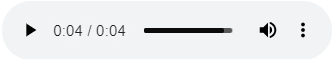## 音频信号数据

``````print ('Example shape ', samples.shape, 'Sample rate ', sample_rate, 'Data type', type(samples))
print (samples[22400:22420])
``````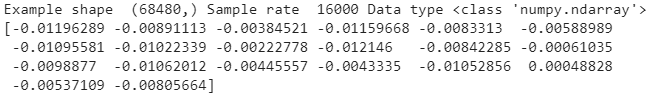## 声谱图

``````sgram = librosa.stft(samples)
librosa.display.specshow(sgram)

``````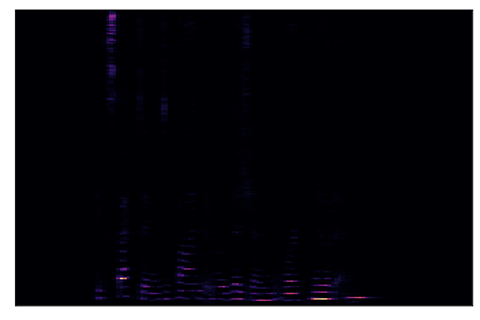## 人类是怎么感知频率的？

• 100 赫兹和 200 赫兹
• 1000 赫兹和1100 赫兹
• 10000 赫兹和10100 赫兹

## 梅尔刻度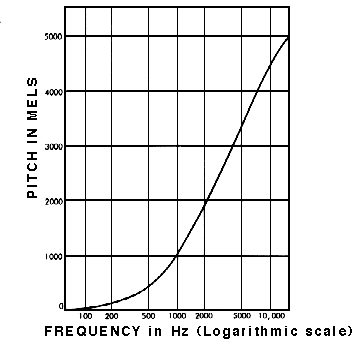## 分贝刻度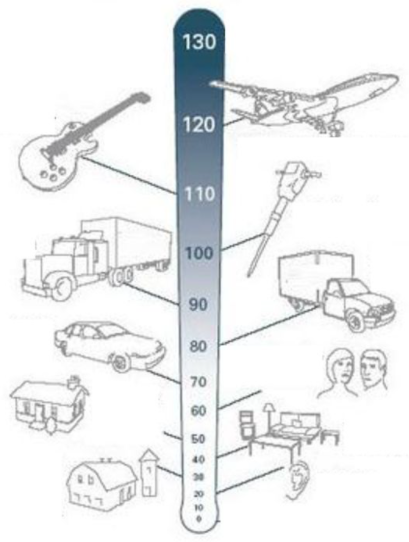## 梅尔声谱图

• Y 轴为梅尔刻度而不是频率。
• 使用分贝刻度代替振幅来指示颜色。

``````# use the mel-scale instead of raw frequency
sgram_mag, _ = librosa.magphase(sgram)
mel_scale_sgram = librosa.feature.melspectrogram(S=sgram_mag, sr=sample_rate)
librosa.display.specshow(mel_scale_sgram)
``````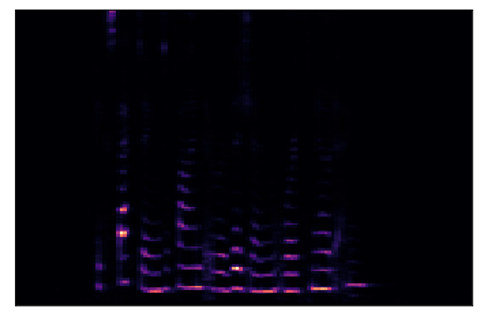``````# use the decibel scale to get the final Mel Spectrogram
mel_sgram = librosa.amplitude_to_db(mel_scale_sgram, ref=np.min)
librosa.display.specshow(mel_sgram, sr=sample_rate, x_axis='time', y_axis='mel')
plt.colorbar(format='%+2.0f dB')
``````